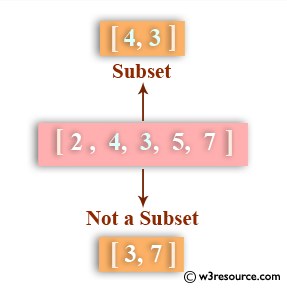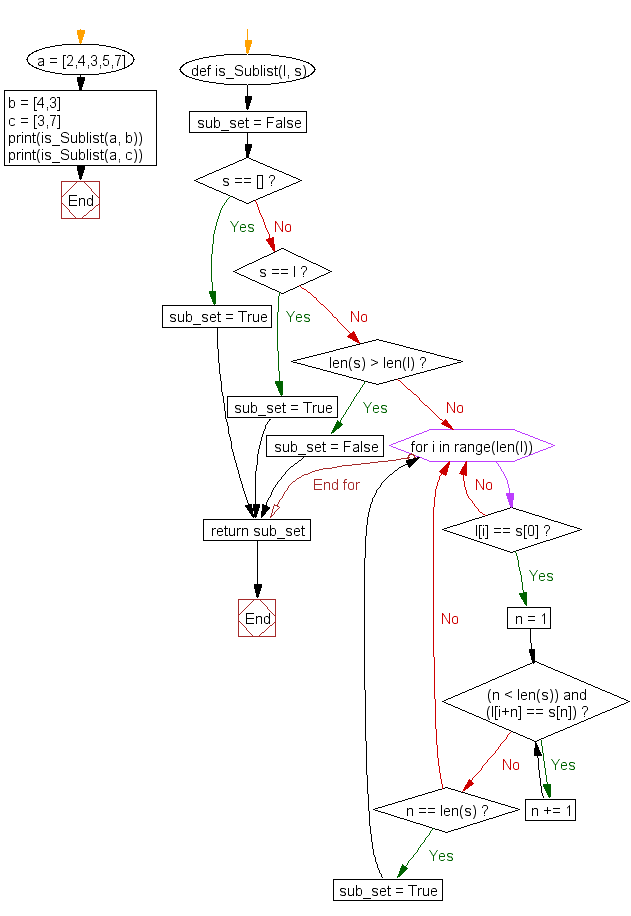﻿ Python: Check whether a list contains a sublist - w3resource# Python: Check whether a list contains a sublist

## Python List: Exercise - 32 with Solution

Write a Python program to check whether a list contains a sublist.Sample Solution:-

Python Code:

``````def is_Sublist(l, s):
sub_set = False
if s == []:
sub_set = True
elif s == l:
sub_set = True
elif len(s) > len(l):
sub_set = False

else:
for i in range(len(l)):
if l[i] == s:
n = 1
while (n < len(s)) and (l[i+n] == s[n]):
n += 1

if n == len(s):
sub_set = True

return sub_set

a = [2,4,3,5,7]
b = [4,3]
c = [3,7]
print(is_Sublist(a, b))
print(is_Sublist(a, c))
```
```

Sample Output:

```True
False
```

Flowchart:## Visualize Python code execution:

The following tool visualize what the computer is doing step-by-step as it executes the said program:

Python Code Editor:

Have another way to solve this solution? Contribute your code (and comments) through Disqus.

What is the difficulty level of this exercise?

Test your Python skills with w3resource's quiz

﻿

## Python: Tips of the Day

Floor Division:

When we speak of division we normally mean (/) float division operator, this will give a precise result in float format with decimals.

For a rounded integer result there is (//) floor division operator in Python. Floor division will only give integer results that are round numbers.

```print(1000 // 300)
print(1000 / 300)```

Output:

```3
3.3333333333333335```